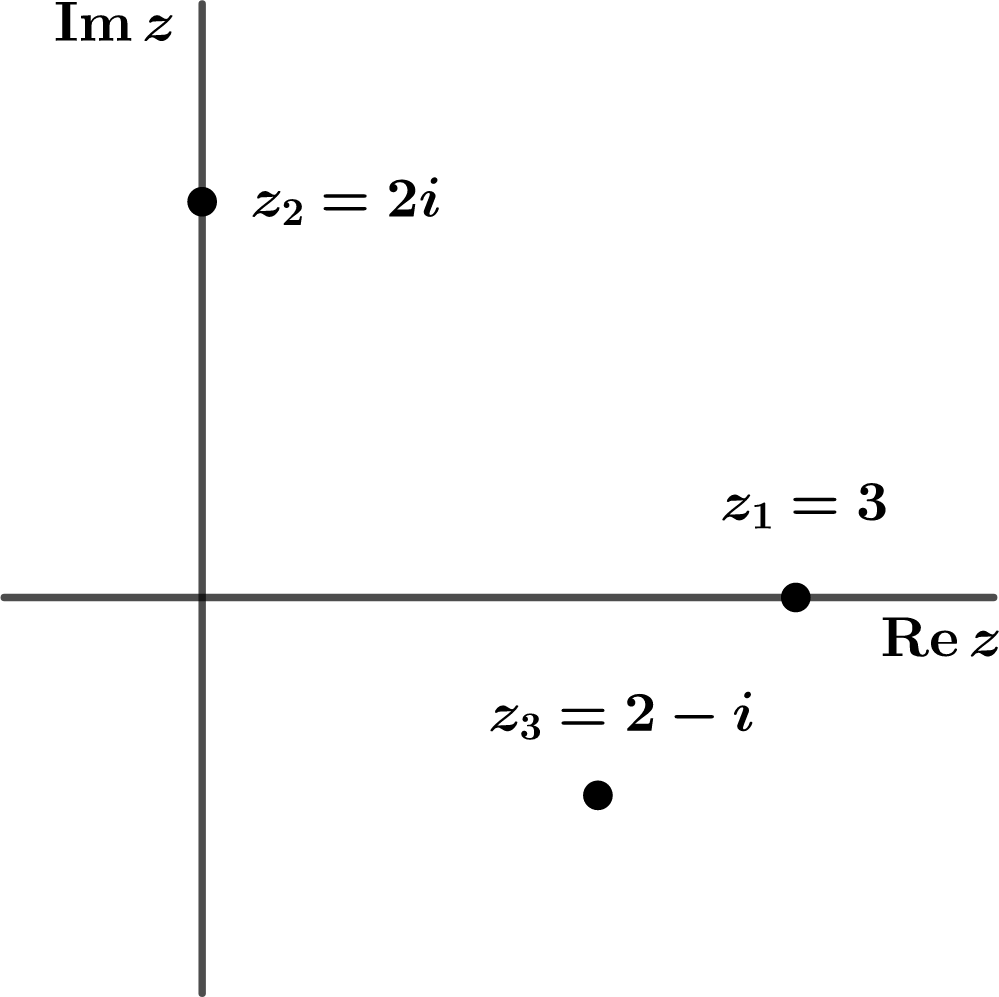## Section2.1The Complex Plane

A complex number $$z$$ is an ordered pair of real numbers $$x$$ and $$y$$ which are distinguished from each other by adjoining the symbol $$i$$ to the second number:

\begin{equation} z=x+iy\tag{2.1.1} \end{equation}

The first number, $$x$$ is called the real part of the complex number $$z$$ and the second number, $$y$$ is called the imaginary part of $$z\text{.}$$ Notice that the imaginary part of $$z$$ is a REAL number.

It is helpful to graph a real number on the real number line. Similarly, it can be helpful to graph a complex number on a 2-d plane where $$x$$ is recorded on the horizontal axis (called the real axis) and $$y$$ is recorded on the vertical axis (called the imaginary axis). This plane, shown in Figure 2.1.1, is called the complex plane or, sometimes, an Argand diagram.Figure 2.1.1. Several complex numbers plotted as points in the complex plane.

A number that lies on the real axis has zero for its imaginary part. Such a number is called real or, for emphasis, pure real. A number that lies on the imaginary axis has zero for its real part. Such a number is called pure imaginary.

There is a powerful analogy between vectors in two dimensions and complex numbers that should be obvious from the graph of the complex plane. Every operation that you can do with two dimensional vectors has an analogue with complex numbers. But the reverse is not true; as you will see in the next section, complex numbers have additional structure that comes from a multiplication rule.